# [Paper Review] Attention is all you need

bona-park·2022년 11월 17일
0

## GDSC-ML

목록 보기
4/5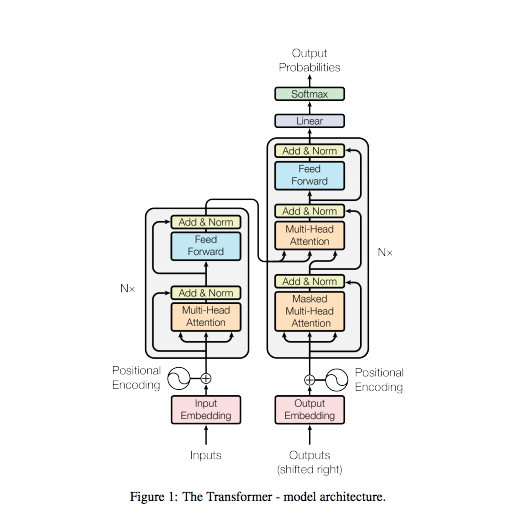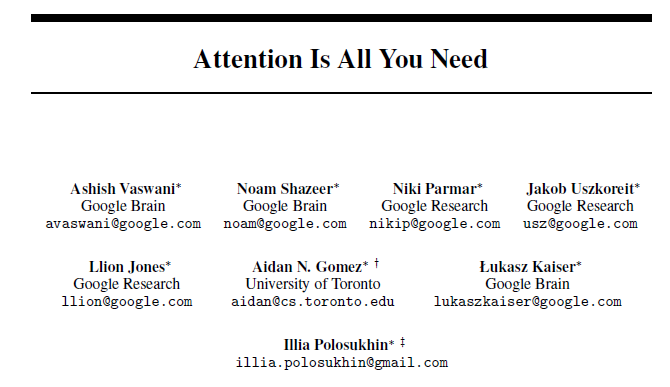### Before..

1. RNN
2. LSTM
: slow to train

Can we parallelize sequential data?

## Transformers

Input sequence can be transmitted parallel

No concept of time step

Pass all the words simultaneously and determine the word embedding simultaneously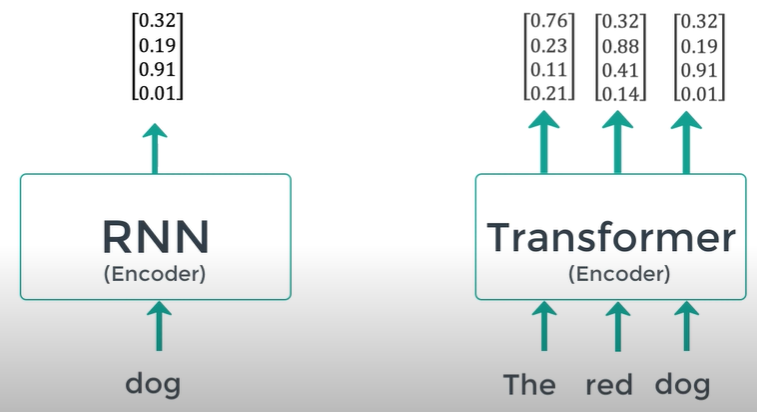(RNN passes input word one after another)

### Input Embedding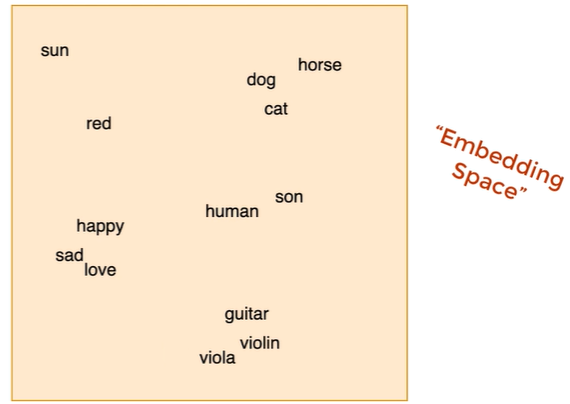In embedding space, close-meaning words locates close to each other

But, same word in a different sentence has a different meaning!

### Positional Encoder

: vector that gives context information based on position of word in a sentence

Can use sin/cos function to generate PE, but any reasonable function is ok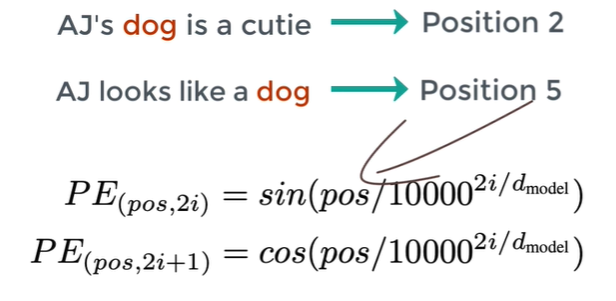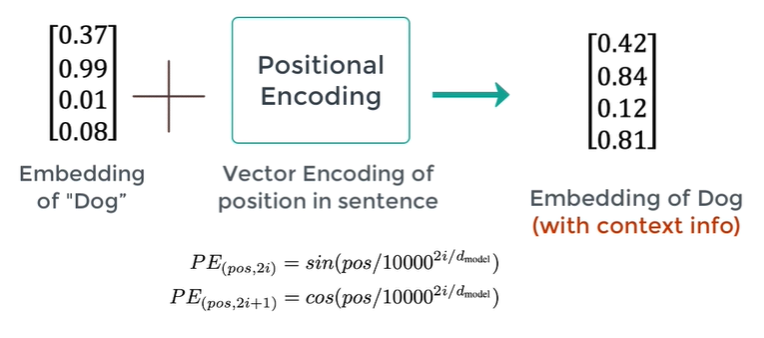## Structure of Encoder

### 1. Attention

: What part of the input should we focus?

How much the word 'The' is relevant to other words(big, red, dog) in the same sentence?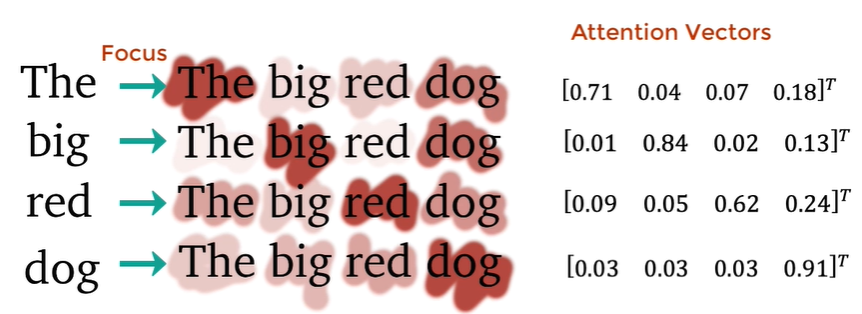Attention Vetors(of English) contain contextual relationships betweeen the words in the sentence.

### 2. Feed Forward

Simple feed forward network is applied to every one of the attention vectors!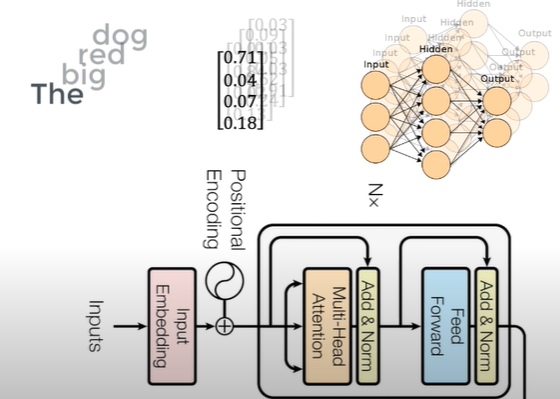#### Problem of Attention vector

Focuses to much on itself..
We want to know the interactions and relationships between words!

Use mulitple attention vector for the same word and average them: Multi Head Attention Block
(Q. vectors from different sentence..?)

Attention vectors are feeded to Feed Forward Network one vector at a time
Each of the attention vector of different word is independent each other
➡ can Parallize Feed Forwared Network!
➡ All words can be passed to the encoder block at the same time and output is a set of encoded vectors for every word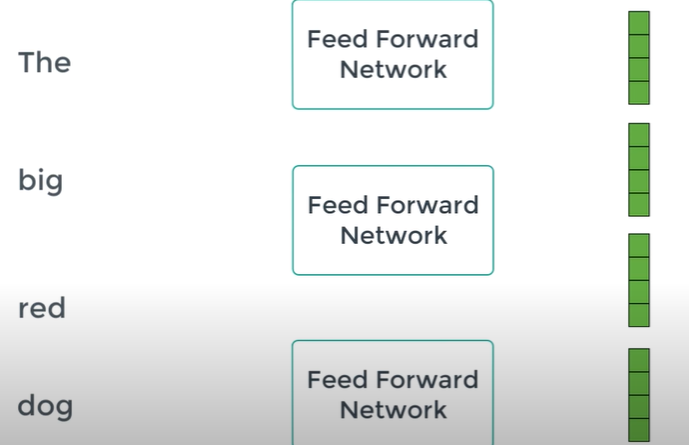## Structure of Decoder

In English -> French task, we feed output French to the decoder

### 1. Self attention block

Generates attention vectors(of French) showing how much each word is related to another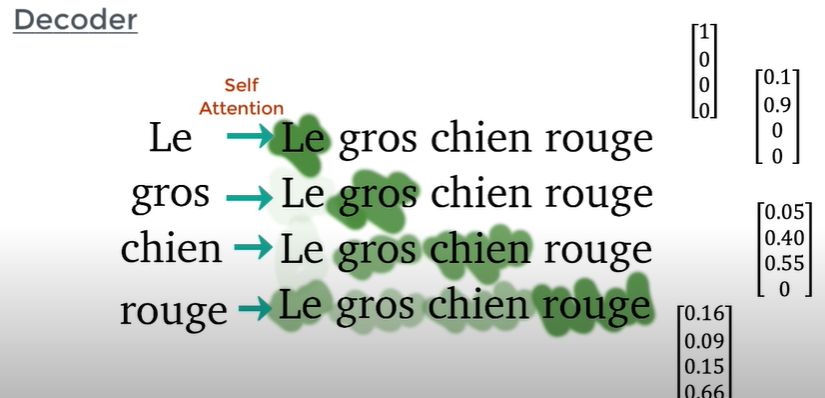Attention vectors from both Encoder(English) and Decoder(French) are passed to another Encoder-Decoder Attention block.
➡ output of this block: Attention vector of all words(English+French)
➡ Each attention vector shows the realtionship of other words including both languages
➡ English to French word mapping happens!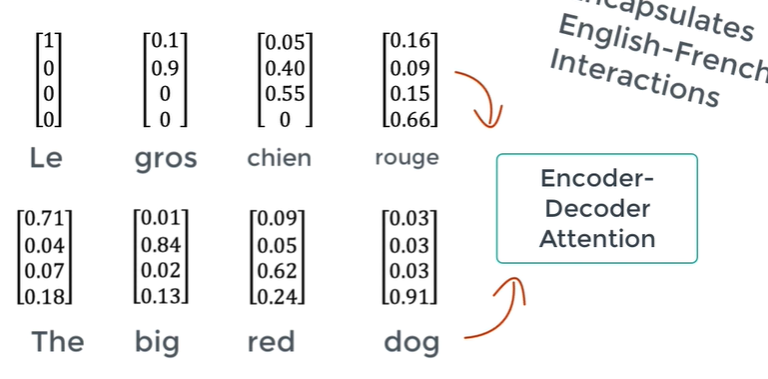If we use all the words in the French sentence, there'd be no learning, just spitting out the next word
mask input: observe only previous and itself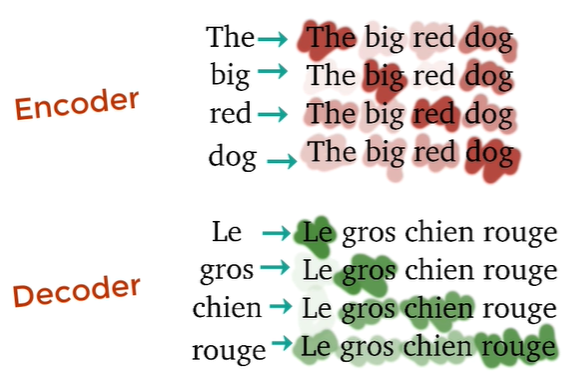V,K,Q: abstract vector that extracts different components of input words
We have V,K,Q vectors for every single word
➡ create attention vector for every word using V, K, Q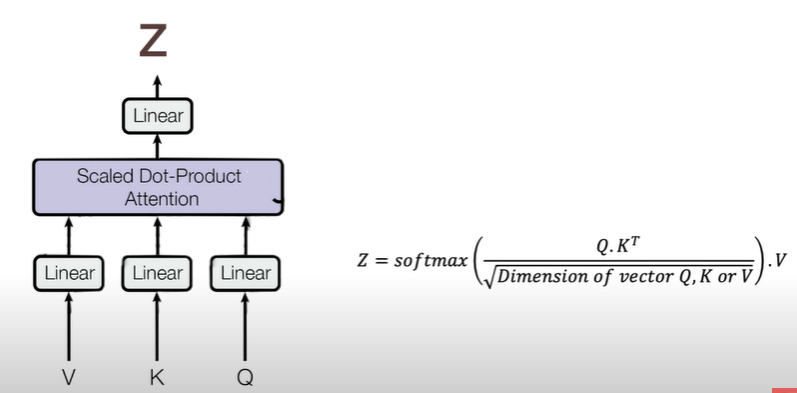Have multiple weight matrices(Wv, Wk, Wq)
➡ multiple attention vectors for every word
➡ another weighted matrices(Wz)
➡ now feed forward nn can be fed only one attention vector per word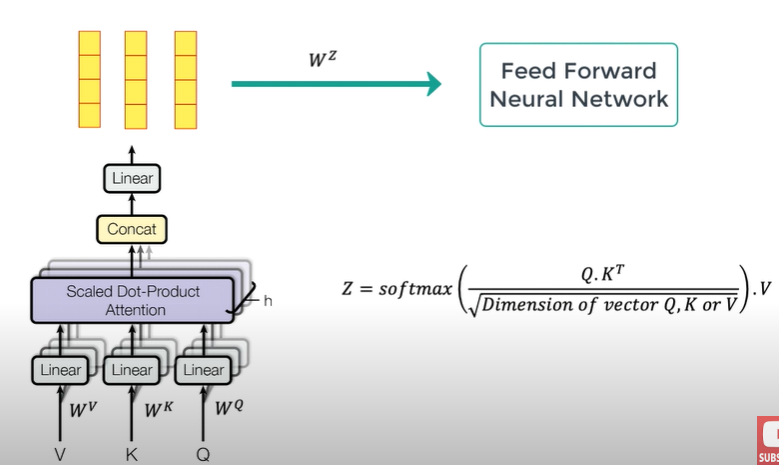### 2. Feed forward unit

Pass each attention vector to feed forward unit

### 3. Linear layer

: another Feed Forward Layer

Used to expand the dimension to the number of words in French

### 4. Softmax layer

Transforms it into Probability Distribution

Output: The word with the highest probability to come next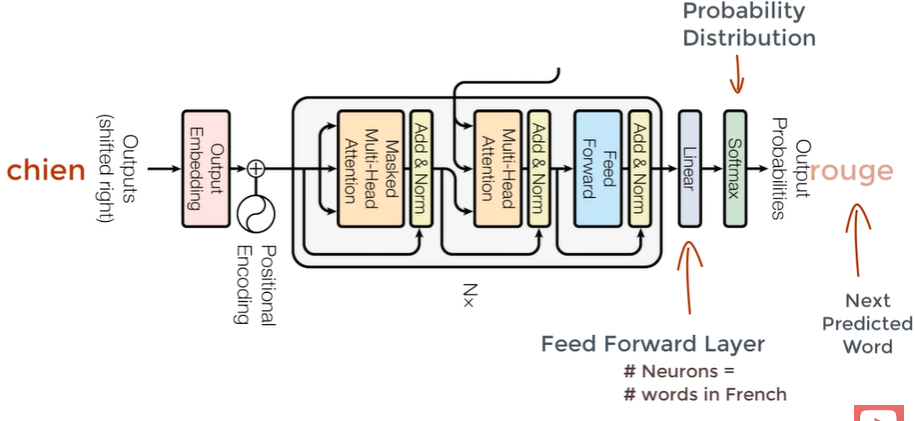### Codes

Reference to https://github.com/hyunwoongko/transformer

Scale Dot Production Attention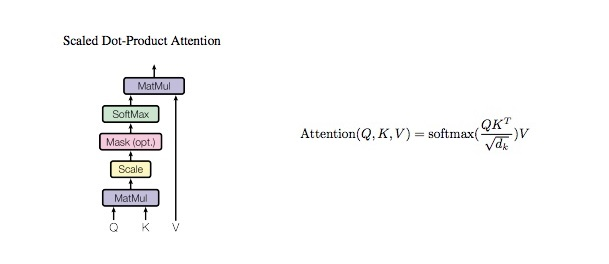class ScaleDotProductAttention(nn.Module):
"""
compute scale dot product attention

Query : given sentence that we focused on (decoder)
Key : every sentence to check relationship with Qeury(encoder)
Value : every sentence same with Key (encoder)
"""

def __init__(self):
super(ScaleDotProductAttention, self).__init__()
self.softmax = nn.Softmax(dim=-1)

def forward(self, q, k, v, mask=None, e=1e-12):
# input is 4 dimension tensor
batch_size, head, length, d_tensor = k.size()

# 1. dot product Query with Key^T to compute similarity
k_t = k.transpose(2, 3)  # transpose
score = (q @ k_t) / math.sqrt(d_tensor)  # scaled dot product

# 3. pass them softmax to make [0, 1] range
score = self.softmax(score)

# 4. multiply with Value
v = score @ v

return v, score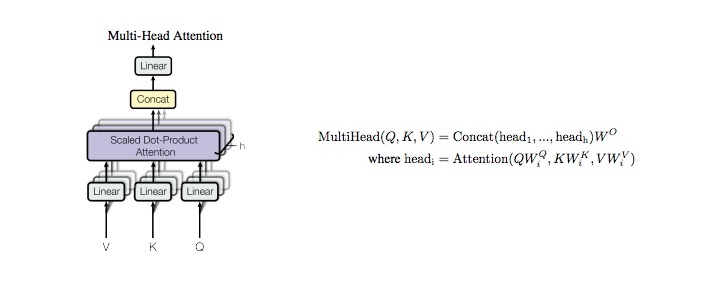class MultiHeadAttention(nn.Module):

self.attention = ScaleDotProductAttention()
self.w_q = nn.Linear(d_model, d_model)
self.w_k = nn.Linear(d_model, d_model)
self.w_v = nn.Linear(d_model, d_model)
self.w_concat = nn.Linear(d_model, d_model)

def forward(self, q, k, v, mask=None):
# 1. dot product with weight matrices
q, k, v = self.w_q(q), self.w_k(k), self.w_v(v)

# 2. split tensor by number of heads
q, k, v = self.split(q), self.split(k), self.split(v)

# 3. do scale dot product to compute similarity

# 4. concat and pass to linear layer
out = self.concat(out)
out = self.w_concat(out)

# 5. visualize attention map
# TODO : we should implement visualization

return out

def split(self, tensor):
"""
split tensor by number of head

:param tensor: [batch_size, length, d_model]
"""
batch_size, length, d_model = tensor.size()

tensor = tensor.view(batch_size, length, self.n_head, d_tensor).transpose(1, 2)
# it is similar with group convolution (split by number of heads)

return tensor

def concat(self, tensor):
"""
inverse function of self.split(tensor : torch.Tensor)

:param tensor: [batch_size, head, length, d_tensor]
:return: [batch_size, length, d_model]
"""
batch_size, head, length, d_tensor = tensor.size()